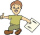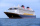# Boys and girls

If the ratio of male & female teachers is 2/5 & it is in proportion of the male & female students, how many are girls if there are 42 boys?

g =  30

### Step-by-step explanation:Did you find an error or inaccuracy? Feel free to write us. Thank you!Tips to related online calculators
Need help to calculate sum, simplify or multiply fractions? Try our fraction calculator.
Check out our ratio calculator.

## Related math problems and questions:

• Boys and girls 8In a class of 35 boys and girls that has a ratio of 2:5, 5 boys are absent and 5 boys are present. How many students are present?
• The ratio 3The ratio of male pupils to female pupils in Gymnasium is 7:9. If there are 512 pupils, how many pupils are male?
• There 12There are 42 students in the class and 2/3 of them are girls. How may girls are there in the class?
• Prospering studentsThere are girls and 30 boys in the class. Boys prospering 28, girls all. How many girls are in the class and how many boys are there, if all the prospering students are 95%?
• ClassroomThere are eighty more girls in the class than boys. Boys are 40 percent and girls are 60 percent. How many are boys and how many girls?
• The ratioThe ratio of girls to boys in Mrs. White's class is 3:2.  If there are 12 boys, how many girls are in the classroom?
• PupilsThere are 32 pupils in the classroom, and girls are two-thirds more than boys. a) How many percents are more girls than boys? Round the result to a whole percentage. b) How many boys are in the class? c) Find the ratio of boys and girls in the class. Writ
• ChildrenThe group has 42 children. There are 4 more boys than girls. How many boys and girls are in the group?
• StudentsThere are 2/6 girls out of 1050 students at the school. Of these, 4/5 have another sibling. How many girls are in school? How many boys are in school? How many girls do not have a sibling?
• A class IV.CIn a class 2/5 were boys. 30 were girls. How many more girls than boys are there?
• Report 2A School reports students to teacher ratio of 6:1. If there are 45 teachers in the School, how many students are there?
• Red diplomasThe numbers of students with honors in 2013 and 2014 are in ratio 40:49. How big is the year-on-year percentage increase?
• Boys and girlsThere are 20 boys and 10 girls in the class. How many different dance pairs can we make of them?
• FortyForty five of the 80 students were girls. What is the ratio of girls to boys?
• Boys and girls 2The ratio of boys to girls in math club is 4:3 . After 8 more girls joined the Club, the ratio become 1:1. How many members are there in the club now?
• Four-member teamThere are 14 girls and 11 boys in the class. How many ways can a four-member team be chosen so that there are exactly two boys in it?
• Boats 2Three quarters of the boats in the marina are white 4/7 of the remaining boats are blue, rest are red if there are 9 red boats. How many boats are in the marina?